Examples

Chapter 2 Class 10 Polynomials
Serial order wise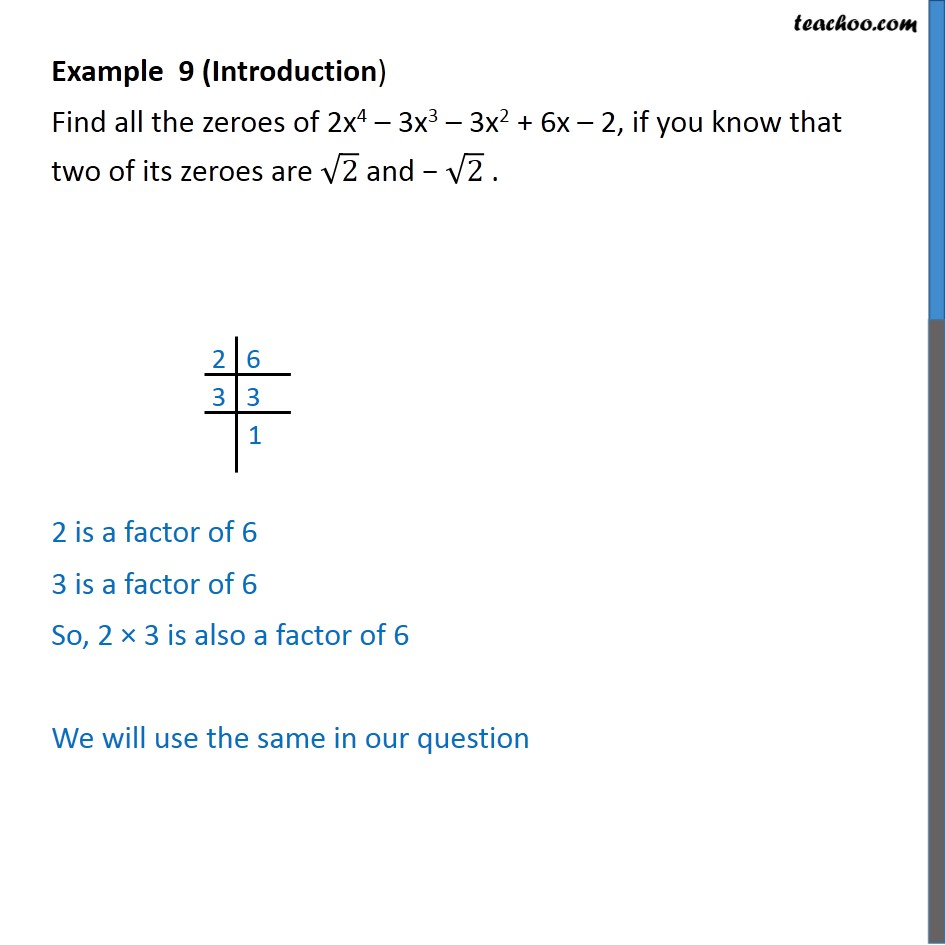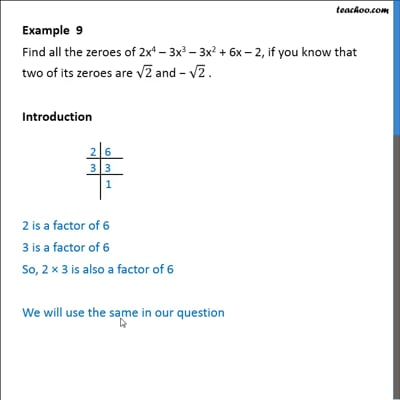This video is only available for Teachoo black users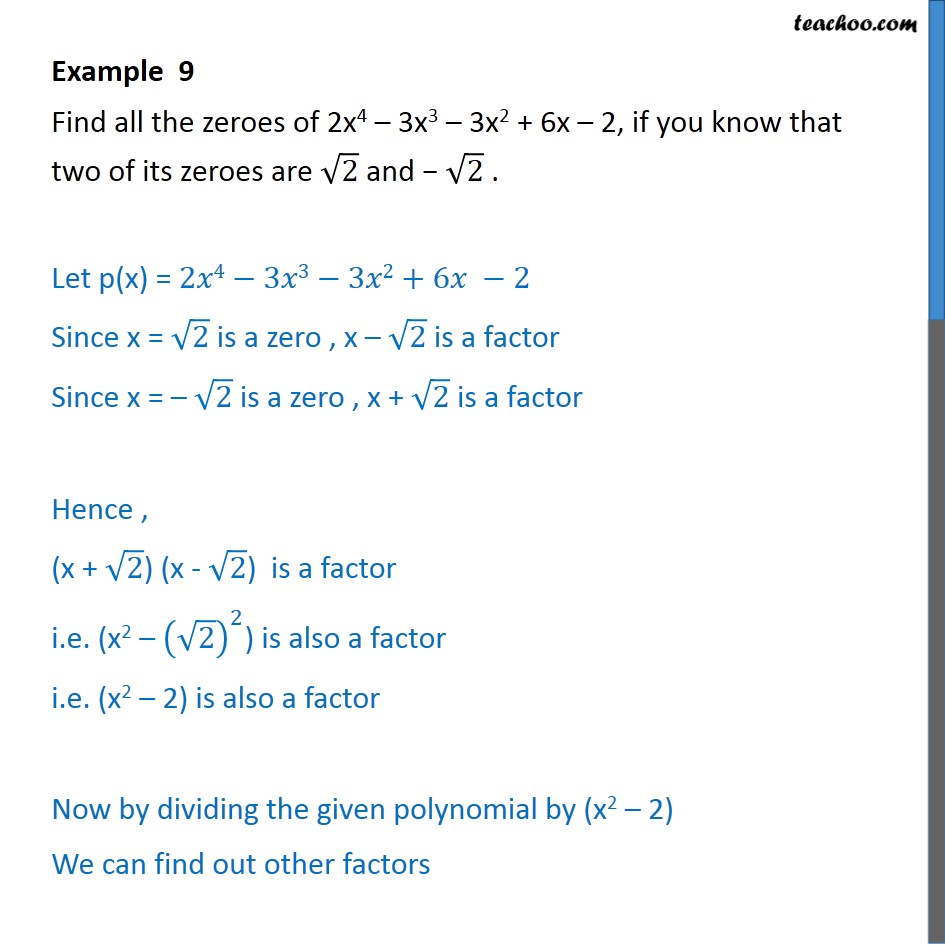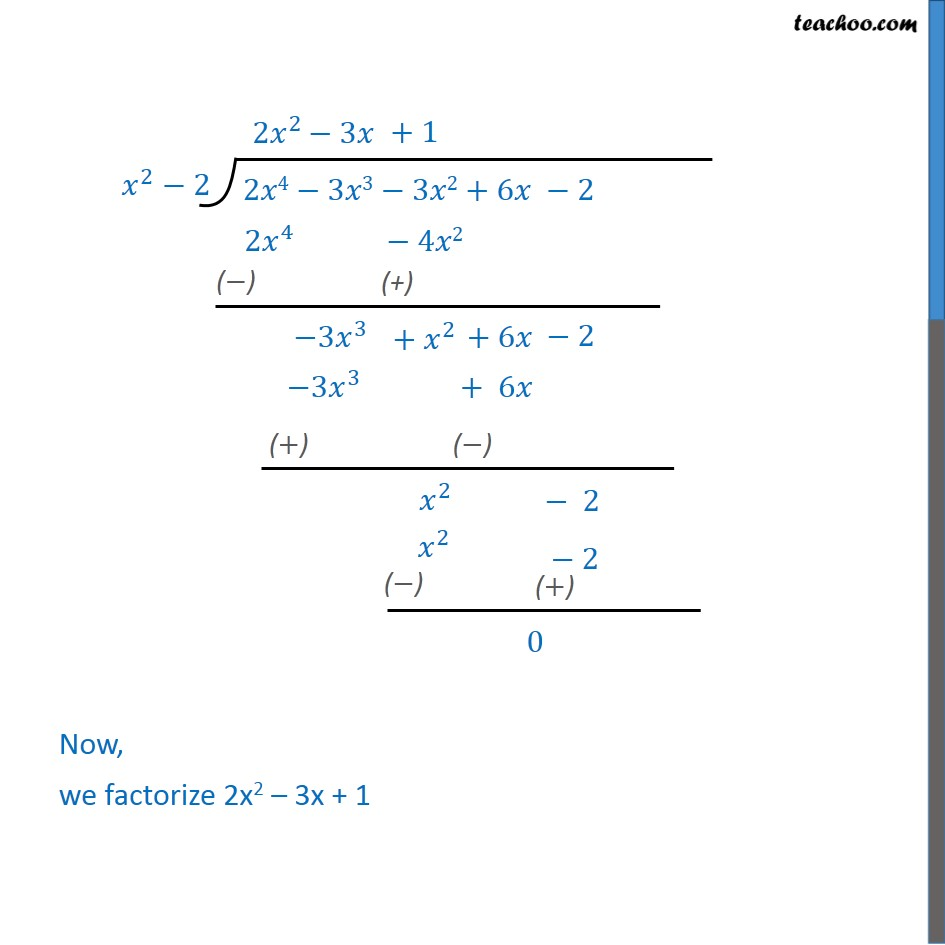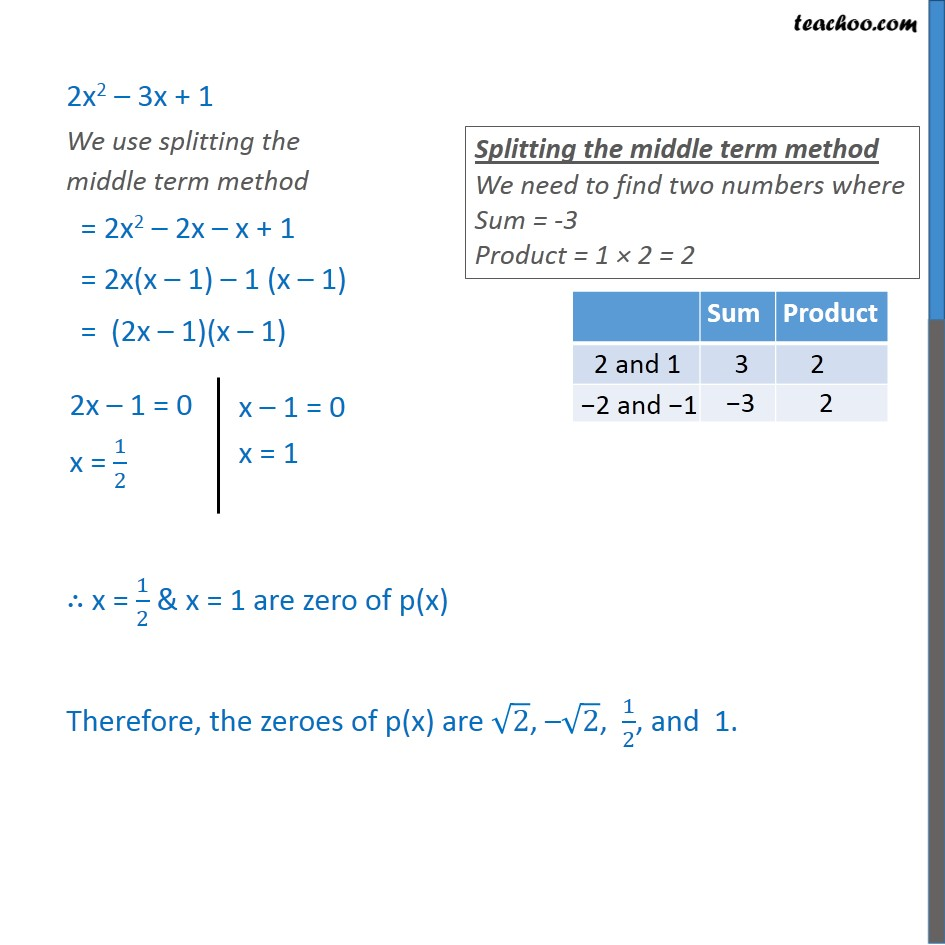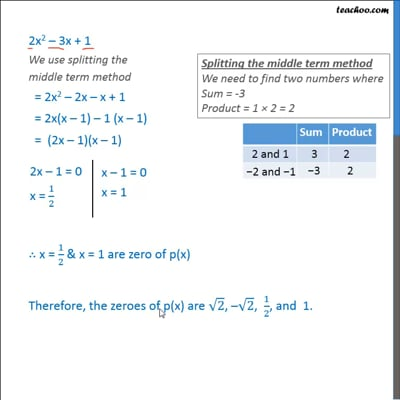This video is only available for Teachoo black users

Solve all your doubts with Teachoo Black (new monthly pack available now!)

### Transcript

Example 9 (Introduction) Find all the zeroes of 2x4 – 3x3 – 3x2 + 6x – 2, if you know that two of its zeroes are √2 and − √2 . 2 is a factor of 6 3 is a factor of 6 So, 2 × 3 is also a factor of 6 We will use the same in our question Example 9 Find all the zeroes of 2x4 – 3x3 – 3x2 + 6x – 2, if you know that two of its zeroes are √2 and − √2 . Let p(x) = 2𝑥4−3𝑥3−3𝑥2+6𝑥 −2 Since x = √2 is a zero , x – √2 is a factor Since x = – √2 is a zero , x + √2 is a factor Hence , (x + √2) (x - √2) is a factor i.e. (x2 – (√2)^2) is also a factor i.e. (x2 – 2) is also a factor Now by dividing the given polynomial by (x2 – 2) We can find out other factors Now, we factorize 2x2 – 3x + 1 2x2 – 3x + 1 We use splitting the middle term method = 2x2 – 2x – x + 1 = 2x(x – 1) – 1 (x – 1) = (2x – 1)(x – 1) ∴ x = 1/2 & x = 1 are zero of p(x) Therefore, the zeroes of p(x) are √2, –√2, 1/2, and 1.